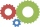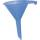# Cube root + exponentiation - math problems

#### Number of problems found: 12

• If the 3If the 6th term of a GP is 4 and the 10th is 4/81, find common ratio r.
• Six speedsA drilling machine is to have 6 speeds ranging from 50 to 750 revolutions per minute. If the speed forms a geometric progression, determine their values.
• Three members GPThe sum of three numbers in GP (geometric progression) is 21 and the sum of their squares is 189. Find the numbers.
• Profit growthThe profit of a company increased by 25% during the year 1992, increased by 40% during the year 1993, decreased by 20% in 1994 and increased by 10% during the year 1995. Find the average growth in the profit level over the four years periods?
• Cube surface and volumeFind the surface of the cube with a volume of 27 dm3.
• Z9-I-4Kate thought a five-digit integer. She wrote the sum of this number and its half at the first line to the workbook. On the second line wrote a total of this number and its one fifth. On the third row, she wrote a sum of this number and its one nines. Fina
• Cube 6Volume of the cube is 216 cm3, calculate its surface area.
• FunnelThe funnel has the shape of an equilateral cone. Calculate the surface wetted with water if we poured into the funnel 7.1 liters of water.
• Prism XThe prism with the edges of the lengths x cm, 2x cm, and 3x cm has volume 20250 cm3. What is the area of the surface of the prism?
• BallsThree metal balls with volumes V1=71 cm3 V2=78 cm3 and V3=64 cm3 melted into one ball. Determine it's surface area.
• PowerNumber left(sqrt(14 * sqrt[ 4 ] (14)) right) 17 can be written in the form 14^x. Find the value of x.
• Cube diagonalDetermine the length of the cube diagonal with edge 37 mm.

We apologize, but in this category are not a lot of examples.
Do you have an exciting math question or word problem that you can't solve? Ask a question or post a math problem, and we can try to solve it.

We will send a solution to your e-mail address. Solved examples are also published here. Please enter the e-mail correctly and check whether you don't have a full mailbox.

Cube root - math problems. Exponentiation - math problems.